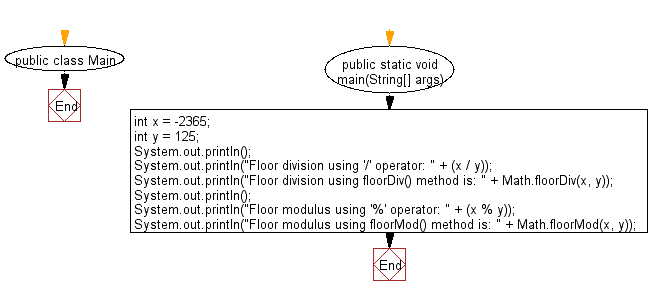﻿ Java exercises: Compute the floor division and the floor modulus of the given dividend and divisor - w3resource# Java Data Type Exercises: Compute the floor division and the floor modulus of the given dividend and divisor

## Java Data Type: Exercise-13 with Solution

Write a Java program to compute the floor division and the floor modulus of the given dividend and divisor.

Sample Solution:

Java Code:

``````public class Main {
public static void main(String[] args) {
int x = -2365;
int y = 125;
System.out.println();
System.out.println("Floor division using '/' operator: " + (x / y));
System.out.println("Floor division using floorDiv() method is: " + Math.floorDiv(x, y));
System.out.println();
System.out.println("Floor modulus using '%' operator: " + (x % y));
System.out.println("Floor modulus using floorMod() method is: " + Math.floorMod(x, y));
}
}
```
```

Sample Output:

```Floor division using '/' operator: -18
Floor division using floorDiv() method is: -19

Floor modulus using '%' operator: -115
Floor modulus using floorMod() method is: 10
```

Flowchart:Java Code Editor:

Improve this sample solution and post your code through Disqus

What is the difficulty level of this exercise?

﻿

## Java: Tips of the Day

Checks if a string is upper case:

```public static boolean isUpperCase(String input) {
return Objects.equals(input, input.toUpperCase());
}
```

Ref: https://bit.ly/39Hpo84